# Solutions: Understanding Percent

#### The Meaning of Percent

 Exercise Problem Solution 1 Which of the following is equal to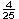? 1.6 8 to 100 16% none of the above 16% 2 Which of the following is equal to 21.8%? 218 to 100 .218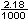none of the above .218 3 Which of the following is equal to 150% 15.0 1.50 .150 None of the above 1.50 4 Which of the following is equal to .0179? 1.79% 17.9% 179% None of the above 1.79% 5 Which of the following is equal to 56.28%? 56.28 5.628 .5628 None of the above .5628

#### Writing Fractions as Percents

 Exercise Problem Solution 1 Which of the following is equal to 36%?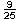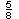None of the above2 Which of the following is equal to 62.5%?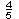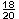None of the above3 Which of the following is equal to.583% 5.83% 58.3% None of the above 58.3% 4 Which of the following is equal to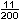? 11% 5.5% 200% None of the above 5.5% 5 What fraction is equal to .42%?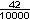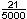All of the above All of the above

#### Writing Decimals as Percents

 Exercise Problem Solution 1 Which of the following is equal to .475? 475% 47.5% 4.75% None of the above 47.5% 2 Which of the following is equal to .0671? .0671% .671% 6.71% None of the above 6.71% 3 Which of the following is equal to .9004? 90.04% 9.004% .9004% None of the above 90.04% 4 What percent of a dollar is a quarter? 2.5% 25% .25% None of the above 25% 5 What percent of a dollar is a nickel? 5% 50% .5% None of the above 5%

#### Writing Percents as Decimals

 Exercise Problem Solution 1 Which of the following is equal to 64%? .064 .64 6.74 None of the above .64 2 Which of the following is equal to 7.27%? 7.27 .727 .0727 None of the above .0727 3 Which of the following is equal to 91.4763%? .914763 9.14673 91.4763 None of the above .914763 4 Which number has the greatest value? 19.72% 1.972 \$19.72 20 cents \$19.72 5 Which number has the least value? 1.039% 1.39 \$1.39 139 cents 1.039%

#### Writing Percents as Fractions

 Exercise Problem Solution 1 Which of the following is equal to 90%?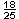None of the above2 Which of the following is equal to 16%?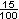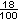None of the above3 Which of the following is equal to? .72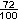72% All of the above All of the above 4 Which of the following is equal to .875? 8.75%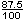None of the above5 Which number has the greatest value? .625 6.25% \$6.25 6,250 cents 6,250 cents

#### Percents Less Than 1 or Greater Than 100

 Exercise Problem Solution 1 Which of the following is equal to 190%? 190.0 19.0 1.90 None of the above 1.90 2 Which of the following is equal to 0.37%? 0.0037 0.037 0.37 None of the above 0.0037 3 Which of the following is equal to 0.00319? 3.19% 0.319% 0.0319% None of the above 0.319% 4 Which of the following is equal to 29.348? 2,934.8% 293.48% 29.348% None of the above 2,934.8% 5 Which number has the least value? 0.0056 0.56%All have the same value All have the same value

#### Practice Exercises

 Exercise Problem Solution 1 Which of the following is equal to? 4% 16% 25% None of the above 16% 2 Which of the following is equal to 0.218 ? .218% 2.18% 21.8% None of the above 21.8% 3 Which of the following is equal to 95% ?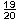None of the above4 Which of the following is equal to 12.5% ? 125 12.5 1.25 None of the above None of the above 5 Which of the following is equal to 62.5% ?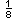None of the above6 Which of the following is equal to 1.75 ? 1.75% 17.5% 175% None of the above 175% 7 Which of the following is equal to?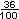0.36 36% All of the above All of the above 8 Which of the following is equal to 0.0947 ? 94.7% 9.47% 0.974% None of the above 9.47% 9 Which of the following is equal to 279% ? 2.79 27.9 279 None of the above 2.79 10 Which of the following is equal to 0.9625% ?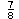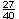None of the above None of the above (0.9625% = 77/8000)

#### Challenge Exercises: Percent Conversions

 Fraction Decimal Percent0.875 87.5%0.95% 95% 18/25 0.72 72%0.0056 0.56% 1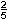1.4 140% 33/200 0.165 16.5% 11/200 0.055 5.5%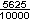0.5625 56.25%0.0042 0.42%